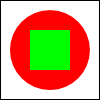[ Quiz Answers | Chapter Index | Main Index ]

## Quiz on Chapter 6

This page contains questions on Chapter 6 of Introduction to Programming Using Java. You should be able to answer these questions after studying that chapter. Sample answers to these questions can be found here.

Question 1:

Programs written for a graphical user interface have to deal with "events." Explain what is meant by the term event. Give at least two different examples of events, and discuss how a program might respond to those events.

Question 2:

A central concept in JavaFX is the scene graph? What is a scene graph?

Question 3:

JavaFX has standard classes called Canvas and GraphicsContext. Explain the purpose of these classes.

Question 4:

Suppose that canvas is a variable of type Canvas. Write a code segment that will draw a green square centered inside a red circle on the canvas, as illustrated.Question 5:

Draw the picture that will be produced by the following for loop, where g is a variable of type GraphicsContext:

```for (int i=10; i <= 210; i = i + 50) {
for (int j = 10; j <= 210; j = j + 50) {
g.strokeLine(i,10,j,60);
}
}```

Question 6:

JavaFX has a standard class called MouseEvent. What is the purpose of this class? What does an object of type MouseEvent do?

Question 7:

Explain the difference between a KeyPressed event and a KeyTyped event.

Question 8:

Explain how AnimationTimers are used to do animation.

Question 9:

What is a CheckBox and how is it used? How does it differ from a RadioButton?

Question 10:

What is meant by layout of GUI components? Explain briefly how layout is done in JavaFX.

Question 11:

How does a BorderPane arrange the components that it contains?

Question 12:

How is the preferred size of a component set, and how is it used?

[ Quiz Answers | Chapter Index | Main Index ]